Ex 3.4

Chapter 3 Class 10 Pair of Linear Equations in Two Variables
Serial order wise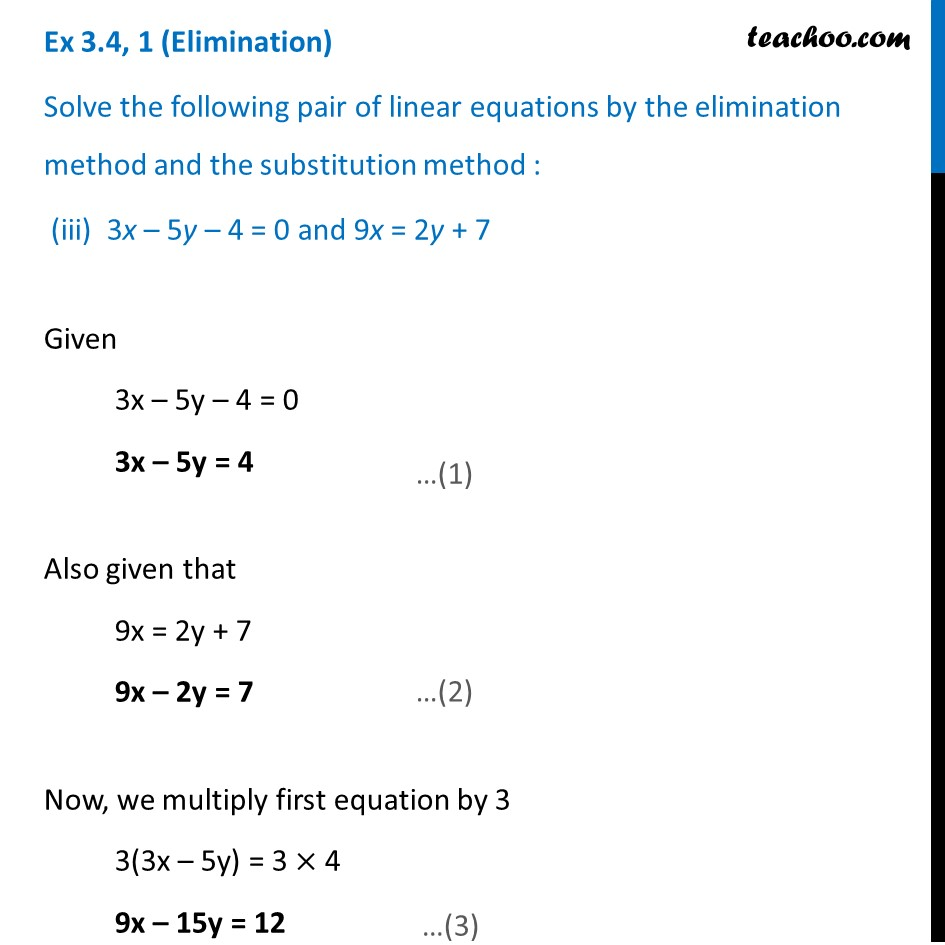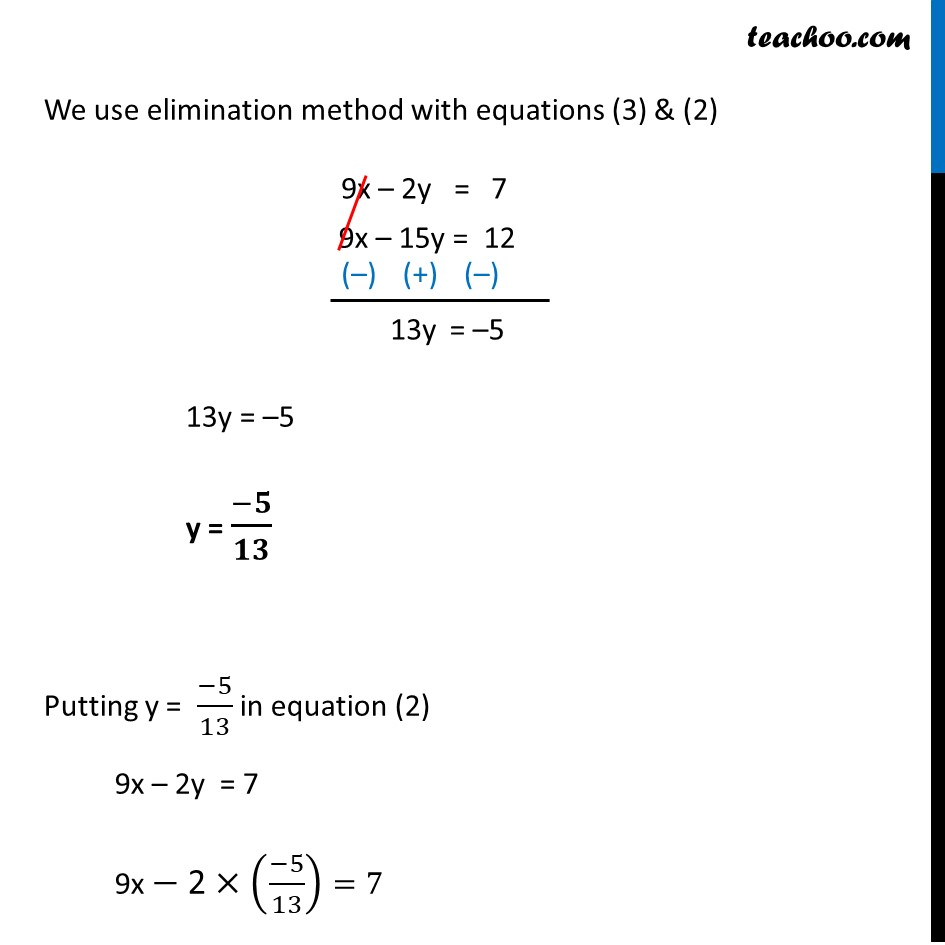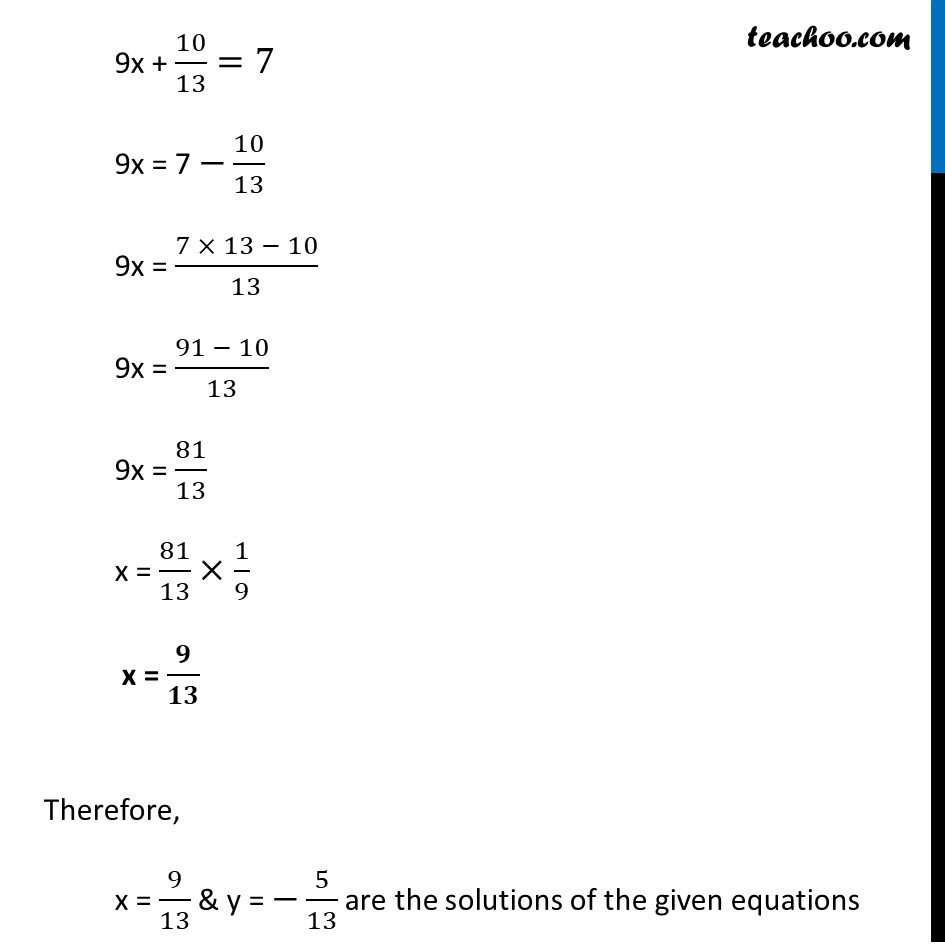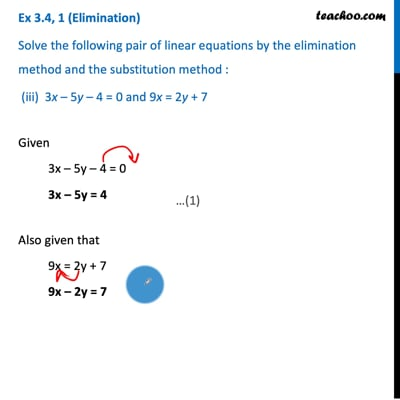This video is only available for Teachoo black users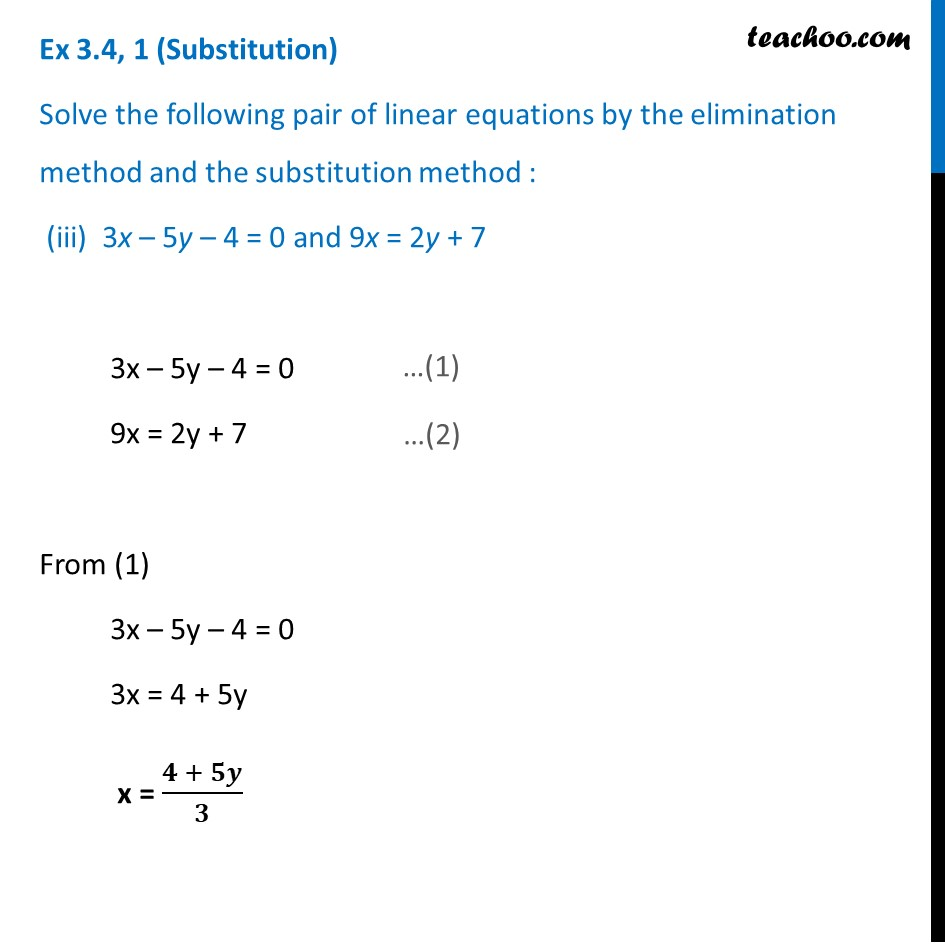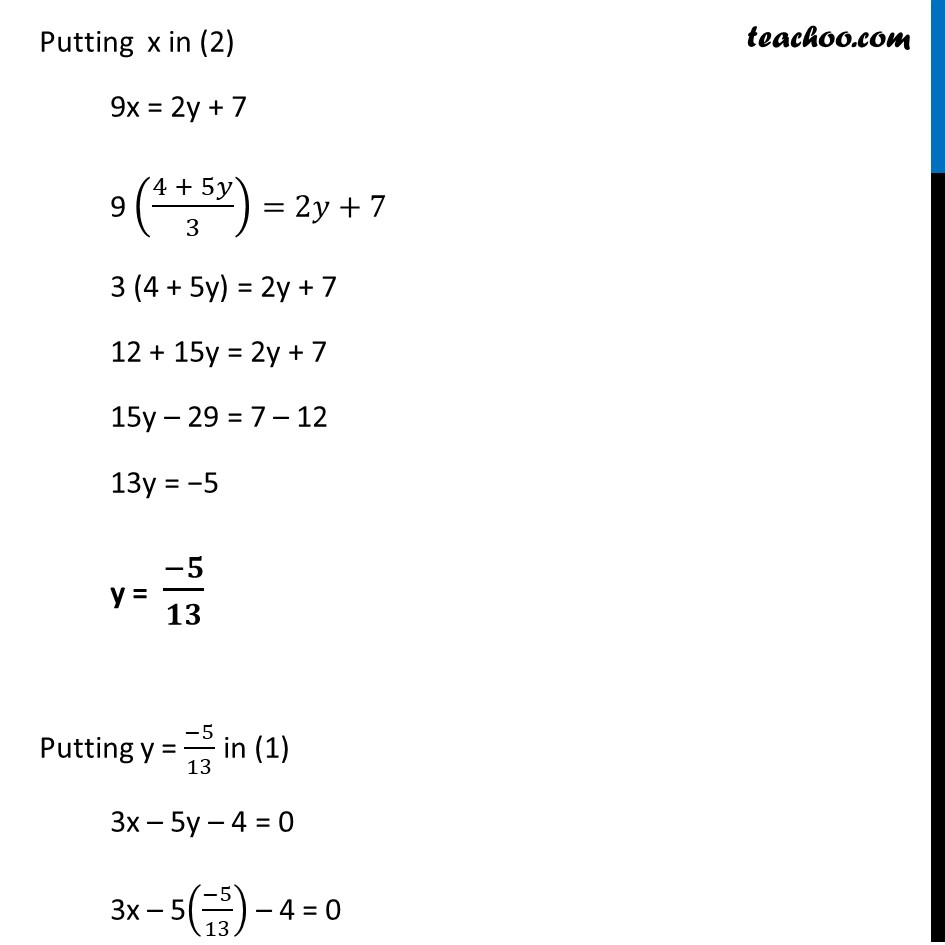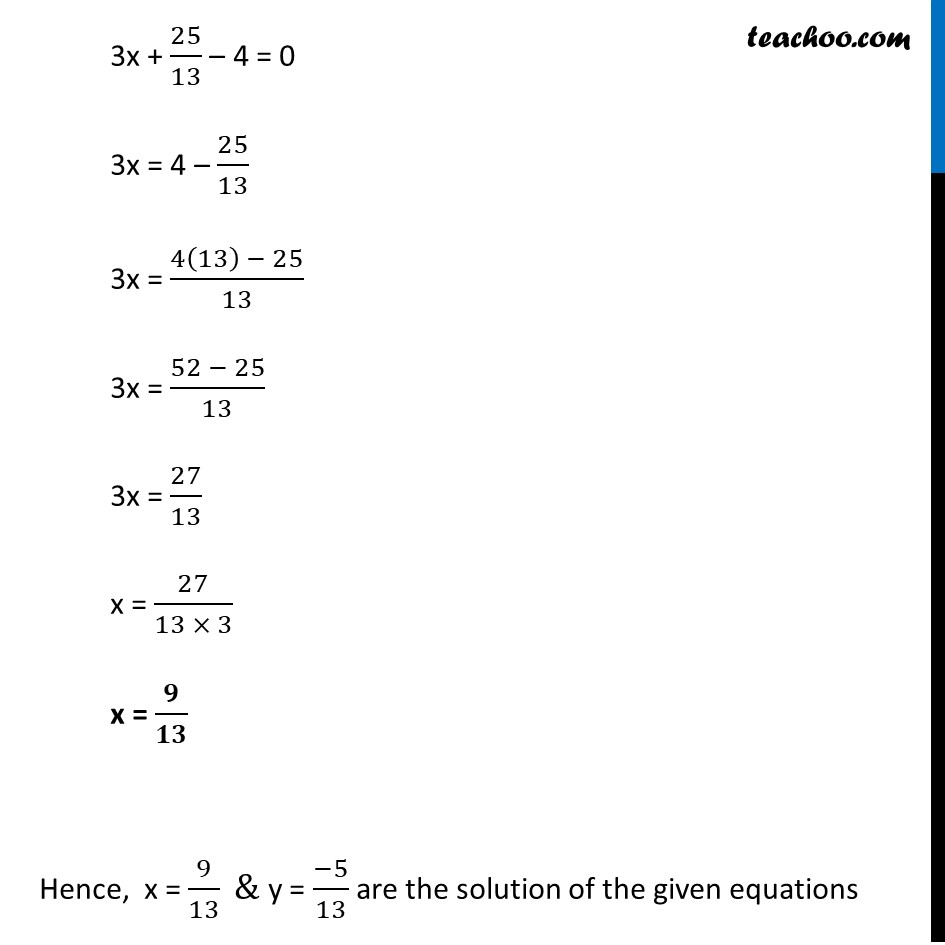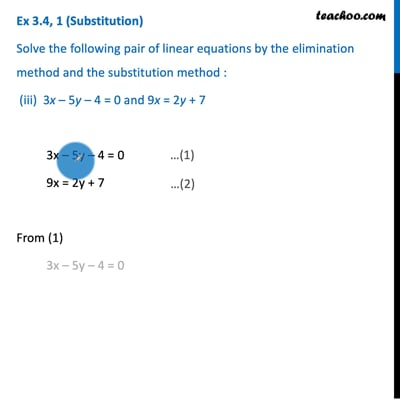This video is only available for Teachoo black users

Introducing your new favourite teacher - Teachoo Black, at only ₹83 per month

### Transcript

Ex 3.4, 1 (Elimination) Solve the following pair of linear equations by the elimination method and the substitution method : (iii) 3x – 5y – 4 = 0 and 9x = 2y + 7 Given 3x – 5y – 4 = 0 3x – 5y = 4 Also given that 9x = 2y + 7 9x – 2y = 7 Now, we multiply first equation by 3 3(3x – 5y) = 3 × 4 9x – 15y = 12 We use elimination method with equations (3) & (2) 13y = –5 y = (−𝟓)/𝟏𝟑 Putting y = (−5)/13 in equation (2) 9x – 2y = 7 9x −" 2"×((−5)/13)=7 9x + 10/13=7 9x = 7 −10/13 9x = (7 × 13 − 10)/13 9x = (91 − 10)/13 9x = 81/13 x = 81/13×1/9 x = 𝟗/𝟏𝟑 Therefore, x = 9/13 & y = −5/13 are the solutions of the given equations Ex 3.4, 1 (Substitution) Solve the following pair of linear equations by the elimination method and the substitution method : (iii) 3x – 5y – 4 = 0 and 9x = 2y + 7 3x – 5y – 4 = 0 9x = 2y + 7 From (1) 3x – 5y – 4 = 0 3x = 4 + 5y x = (𝟒 + 𝟓𝒚)/𝟑 Putting x in (2) 9x = 2y + 7 9 ((4 + 5𝑦)/3)=2𝑦+7 3 (4 + 5y) = 2y + 7 12 + 15y = 2y + 7 15y – 29 = 7 – 12 13y = −5 y = (−𝟓)/𝟏𝟑 Putting y = (−5)/13 in (1) 3x – 5y – 4 = 0 3x – 5((−5)/13) – 4 = 0 3x + 25/13 – 4 = 0 3x = 4 – 25/13 3x = (4(13) − 25)/13 3x = (52 − 25)/13 3x = 27/13 x = 27/(13 × 3) x = 𝟗/𝟏𝟑 Hence, x = 9/13 & y = (−5)/13 are the solution of the given equations